###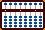Hexadecimal on the Soroban

Used in mathematics and computer sciences, hexadecimal or "hex" is a base 16 numerical system. It's main purpose is to represent the binary code in a way that makes it easier for most of us to read. In fact it acts as a kind of shorthand. In hexadecimal the numbers 0-9 are represented normally then; A=ten, B=eleven, C=twelve, D=thirteen, E=fourteen and F=fifteen.

 0 hex = 0 dec    1 hex = 1 dec  2 hex = 2 dec  3 hex = 3 dec 0  0  0  0   0  0  0  1   0  0  1  0   0  0  1  1 4 hex = 4 dec  5 hex = 5 dec  6 hex = 6 dec  7 hex = 7 dec 0  1  0  0   0  1  0  1   0  1  1  0   0  1  1  1 8 hex = 8 dec  9 hex = 9 dec A hex = 10 dec B hex = 11 dec 1  0  0  0   1  0  0  1   1  0  1  0   1  0  1  1 C hex = 12 dec D hex = 13 dec E hex = 14 dec F hex = 15 dec 1  1  0  0   1  1  0  1   1  1  1  0   1  1  1  1
 Conversion Table

#### Binary Codes

A "binary code" is any system that uses only two states (0 or 1, on or off, true or false, etc.) The base 2 or binary number system is one example of a binary code.

#### How it works for numbers

Take the example 201, which can also be expressed as:  128 + 64 + 8 + 1

```128 = 10000000
64 =  1000000
8 =     1000
1 =        1
201 = 11001001```

So 201 has a binary representation of 11001001. The problem is many of us find binary numbers difficult to understand. Converting 201 to hex makes the number more accessible. (see Example:1)

###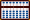Convert Base 10 to Hexadecimal

##### This is an advanced soroban problem using the division techniques taught by Takashi Kojima.

Basically it works like this:

• Designate a unit rod.
• Set the base 10 number onto the soroban.
• Divide by16.
• If the quotient on the left is 16 or greater divide again to make it less than 16.
• Use the Conversion Table to express quotient and remainders as hex.

#### Example 1: Convert 201 to its hexadecimal and binary equivalents

Step 1: Choose rod H as the unit rod. Set 201 and 16 onto the soroban. (Fig.1)Fig.1 `Step 1` ```A B C D E F G H I . . .   1 6 0 0 0 2 0 1 0```

Divide 201 by 16. What remains on the soroban is the quotient 12 & the remainder 09. When expressed as hex 12 & 09 become C9 with a binary representation of 11001001 (C = 1100, 9 = 1001). (Fig.2)Fig.2 `Step 2` ```A B C D E F G H I . . .   1 6 0 0 0 2 0 1 0 (1)   - 1 6   1 6 0 1 0 0 4 1 0 (2)   - 3 2   1 6 0 1 2 0 0 9 0```

#### Example 2: Convert 2989 to its hexadecimal and binary equivalents

Step 1: Choose K as the unit rod. Set 2989 and 16 onto the soroban. (Fig.3)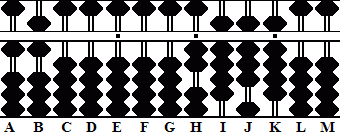Fig.3 `Step 1` ```A B C D E F G H I J K L M . . . .   1 6 0 0 0 0 0 2 9 8 9 0 0```

Step 2: Divide 2989 by 16 leaving the quotient answer 186 on rods FGH. Notice the remainder 13 on rods JK; it forms the last part of the hexadecimal answer. (Fig.4)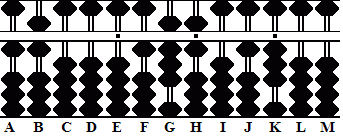Fig.4 `Step 2` ```A B C D E F G H I J K L M . . . .   1 6 0 0 0 0 0 2 9 8 9 0 0 (1)   - 1 6   1 6 0 0 0 1 0 1 3 8 9 0 0 (8)   - 1 2 8   1 6 0 0 0 1 8 0 1 0 9 0 0 (6)   - 9 6   1 6 0 0 0 1 8 6 0 1 3 0 0 ```

Step 3 and the answer: Convert 186 to hex by dividing again. With this last step what remains on the soroban is the quotient 11 and the remainders 10 & 13. When expressed as hex 11, 10 & 13 become BAD with a binary representation of 101110101101 (B = 1011, A = 1010, D = 1101). (Fig.5)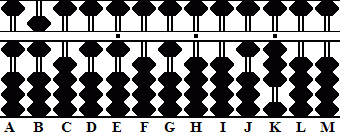Fig.5 `Step 3` ```A B C D E F G H I J K L M . . . .   1 6 0 0 0 1 8 6 0 1 3 0 0 (1)   - 1 6   1 6 0 0 0 0 2 6 0 1 3 0 0 (1)   - 1 6   1 6 0 1 1 0 1 0 0 1 3 0 0```

###Convert Hexadecimal to Base 10

##### This is an advanced soroban problem using the multiplication techniques taught byTakashi Kojima.

The technique for converting hexadecimal to base 10 is very much as the one above but in reverse. In other words instead of dividing we multiply in the reverse order.

• Where applicable use the Conversion Table to express the hexadecimal in numbers.
• Set numbers onto the soroban making sure to set unit numbers on unit rods.
• Work left to right starting with the left-hand most group.
• Multiply each group setting the product on the right.
• Move to the next group and multiply again.

All this is best explained by example. It's not at all complicated. Here's a simple example first.

#### Example 1: Convert the hex D0A to Base 10

Step 1: Expressed as a decimal (base 10) number, hexadecimal D0A becomes 13 00 10. Set each group of numbers in such a way that each unit number falls on a unit rod. In this case 13 on rods GH, 00 on JK & 10 on MN. Set the multiplier 16 on rods AB.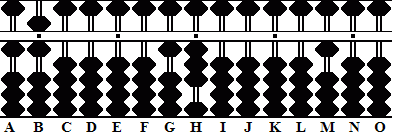Fig.6 `Step 1` ```A B C D E F G H I J K L M N O . . . . .   1 6 0 0 0 0 1 3 0 0 0 0 1 0 0```

Step 2: Starting with the left most group multiply 3 on H by 16. Add the product 48 to rods JK. Clear 3 from H.

2a: Multiply 1 on rod G by 16. Add the product 16 to rods IJ. Clear 1 from rod G. (Fig.7)Fig.7 `Steps 2 & 2a` ```A B C D E F G H I J K L M N O . . . . .   1 6 0 0 0 0 1 3 0 0 0 0 1 0 0 + 4 8 clear (-3)   1 6 0 0 0 0 1 0 0 4 8 0 0 0 0 + 1 6 clear (-1)   1 6 0 0 0 0 0 0 2 0 8 0 1 0 0 ```

Step 3: Now move right to the next group and multiply 8 on K by 16. Add the product 128 to rods LMN. Clear 8 from K.

3a and the answer: Multiply 2 on I by 16. Add the product 32 to rods KL. Clear 2 from I and that completes the problem. Hexadecimal D0A converts to its base 10 number 3338. (Fig.8)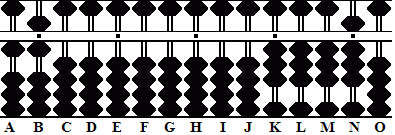Fig.8 `Steps 3 & 3a` ```A B C D E F G H I J K L M N O . . . . .   1 6 0 0 0 0 0 0 2 0 8 0 1 0 0 + 1 2 8 clear (-8)   1 6 0 0 0 2 0 0 2 0 0 1 3 8 0 + 3 2 clear (-2)   1 6 0 0 0 0 0 0 0 0 3 3 3 8 0 ```

#### Example 2: Convert the hex B4E8 to Base 10

Step 1:  Expressed as a decimal (base 10) number, hexadecimal B4E8 becomes 11 04 14 08. As before set each group so that each unit number falls on a unit rod; in this case 11 on DE, 04 on GH, 14 on JK & 08 on MN. (Fig.9)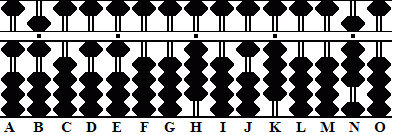Fig.9 `Step 1` ```A B C D E F G H I J K L M N O . . . . .   1 6 0 1 1 0 0 4 0 1 4 0 0 8 0```

Step 2: Starting with the left most multiply 1 on E by16. Add the product 16 to rods GH. Clear 1 from rod E.

2a: Multiply 1 on D by 16. Add the product 16 to rods FG. Clear 1 from rod D. (Fig.10)Fig.10 `Steps 2 & 2a` ```A B C D E F G H I J K L M N O . . . . .   1 6 0 1 1 0 0 4 0 1 4 0 0 8 0 + 1 6 clear (-1)   1 6 0 1 0 0 2 0 0 1 4 0 0 8 0 + 1 6 clear(1)   1 6 0 0 0 1 8 0 0 1 4 0 0 8 0```

Step 3: Now move right to the next group to and multiply 8 on Gt by 16  Add the product 128 to rods HIJ. Clear 8 from G.

3a: Multiply 1 on rod F by 16. Add the product 16 to rods HI. Clear 1 from rod F. (Fig.11)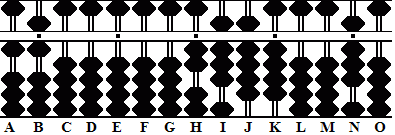Fig.11 `Steps 3 & 3a` ```A B C D E F G H I J K L M N O . . . . .   1 6 0 0 0 1 8 0 0 1 4 0 0 8 0 + 1 2 8 clear (-8)   1 6 0 0 0 1 0 1 2 9 4 0 0 8 0 + 1 6 clear (-1)   1 6 0 0 0 0 0 2 8 9 4 0 0 8 0```

Step 4: Move to the next group and multiply 4 on rod K by 16. Add the product 64 to rods MN. Clear 4 from K.

4a: Multiply 9 on J by 16 and add the product 144  to KLM. Clear 9 from J.

4b: Multiply 8 on rod I by 16, add the product 128 to JKL. Clear 8 from I

4c and the answer: Multiply 2 on on H by16 and add the product 32 to rods IJ. Clear 2 from H and that completes the problem. Hexadecimal B4E8 converts to its base 10 number 46312.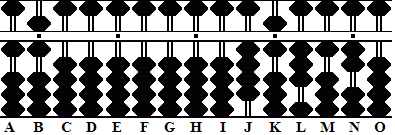Fig.12 `Steps 4 4a 4b & 4c` ```A B C D E F G H I J K L M N O . . . . .   1 6 0 0 0 0 0 2 8 9 4 0 0 8 0 +6 4 clear (-4)   1 6 0 0 0 0 0 2 8 9 0 0 7 2 0 + 1 4 4 clear (-9)   1 6 0 0 0 0 0 2 8 0 1 5 1 2 0 + 1 2 8 clear (-8)   1 6 0 0 0 0 0 2 0 1 4 3 1 2 0 + 3 2 clear (-2)   1 6 0 0 0 0 0 0 0 4 6 3 1 2 0```

####A word of thanks

##### Sincere thanks, Totton Heffelfinger

REFERENCES
:

###### Kojima, Takashi. The Japanese Abacus: Its Use and Theory Tokyo: Charles E. Tuttle, 1954

Print Page (.PDF Format, 108kb)